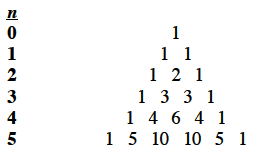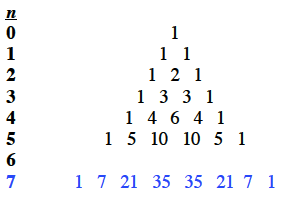### Home > CCA2 > Chapter 10 > Lesson 10.3.1 > Problem10-145

10-145.

Use Pascal’s Triangle to expand $(x + y)^7$.

Recall Pascal's Triangle. Pascal's triangle, up to n, = 5, where the first row is, n, = 0. Last row is 1, 5, 10, 10, 5, 1.
What row is needed to expand $(x + y)^7$?

Use the pattern for $(a + b)^7$. row 7 Added to the triangle with the numbers: 1, 7, 21, 35, 35, 21, 7, 1.$a^7 + 7a^6b + 21a^5b^2 + 35a^4b^3 + 35a^3b^4 + 21a^2b^5 + 7ab^6 + b^7$

Now substitute $x$ for $a$ and $y$ for $b$.